Courses

# Magnetic Field and Biot Savart Law Class 12 Notes | EduRev

## Class 12: Magnetic Field and Biot Savart Law Class 12 Notes | EduRev

The document Magnetic Field and Biot Savart Law Class 12 Notes | EduRev is a part of the Class 12 Course Physics For JEE.
All you need of Class 12 at this link: Class 12

Magnetism:

1. The Magnetic Field:

In earlier lessons we found it convenient to describe the interaction between charged objects in terms of electric fields. Recall that an electric field surrounding an electric charge. The region of space surrounding a moving charge includes a magnetic field in addition to the electric field. A magnetic field also surrounds a magnetic substance.

In order to describe any type of field, we must define its magnitude, or strength, and its direction.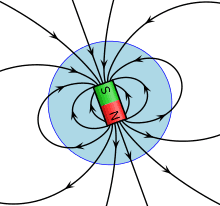Fig: Magnetic field

Magnetic field is the region surrounding a moving charge in which its magnetic effects are perceptible on a moving charge (electric current). Magnetic field intensity is a vector quantity and also known as magnetic induction vector. It is represented by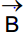Lines of magnetic induction may be drawn in the same way as lines of electric field. The number of lines per unit area crossing a small area perpendicular to the direction of the induction being numerically equal to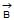. The number of lines of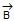crossing a given area is referred to as the magnetic flux linked with that area. For this reasonis also called magnetic flux density.

There are two methods of calculating magnetic field at some point. One is Biot-Savart law which gives the magnetic field due to an infinitesimally small current carrying wire at some point and the another is Ampere' law, which is useful in calculating the magnetic field of a symmetric configuration carrying a steady current.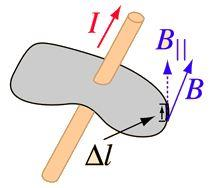Fig: Ampere's law

The unit of magnetic field is weber/m2 and is known as tesla (T) in the SI system.

2. BIOT-SAVART LAW:

Biot-Savart law gives the magnetic induction due to an infinitesimal current element.

Let AB be a conductor of an arbitrary shape carrying a current i, and P be a point in vacuum at which the field is to be determined. Let us divide the conductor into infinitesimal current-elements. Let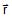be a displacement vector from the element to the point P.

According to `Biot-Savart Law', the magnetic field induction at P due to the current element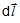is given by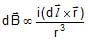or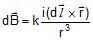Where k is a proportionality constant.

Herevector points in the direction of current i.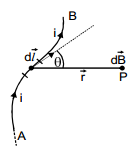In S.I. units,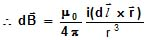...(1)

Equation (1) is the vector form of the Biot-Savart Law. The magnitude of the field induction at P is given by: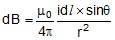,

where θ is the angle betweenand.

If the medium is other than air or vaccum, the magnetic induction is: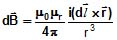...(2)

where μr is relative permeability of the medium and is a dimensionless quantity.

The document Magnetic Field and Biot Savart Law Class 12 Notes | EduRev is a part of the Class 12 Course Physics For JEE.
All you need of Class 12 at this link: Class 12Use Code STAYHOME200 and get INR 200 additional OFF Use Coupon Code
All Tests, Videos & Notes of Class 12: Class 12## Physics For JEE

187 videos|516 docs|263 tests

Track your progress, build streaks, highlight & save important lessons and more!

,

,

,

,

,

,

,

,

,

,

,

,

,

,

,

,

,

,

,

,

,

;